Learning Center > Teaching with Sketchpad > Sample Activities > Elementary Grades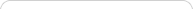##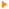Teaching with Sketchpad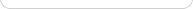•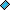- plays a video
•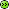- opens a web page##Jump Along: Factor Families on the Number Line

Students investigate factors from a visual perspective as they find all the ways a rabbit can take equal-size jumps to reach a target number. Students write multiplication sentences to represent the rabbit’s jumps, and uncover the commutative property of multiplication.

##Bugs in Groups: Dividing into Groups of Equal Size

Students explore and connect part-whole relationships in multiplication and division as they order a collection of bugs into groups of equal size and consider the number of groups and the number of bugs left over. Concepts of factors, remainders, and the commutative property of multiplication all arise in students’ work with the model.

##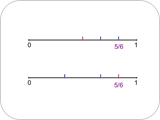Dividing and Subdividing: Fractions on the Number Line

Students locate fractions less than 1 along a number line using virtual tools and a strategy of dividing and subdividing. The challenge is to find more than one way to locate a given fraction.

##Place-Value Counter: Get to the Target

Students use their knowledge of place value and their intuitive notions of rounding to operate an interactive numerical counter that works like an odometer. Using buttons that increase or decrease the value of the counter by one, ten, one hundred, one thousand, or more, students work to reach given target values in as few button presses as possible.

##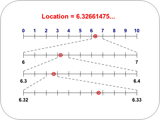Zooming Decimals: Precision and Place Value

Students zoom in on a number line to reveal scales calibrated to tenths, hundredths, thousandths, and ten-thousandths. They reason about decimals and place value as they name with increasing precision the location of a point on the number line.

##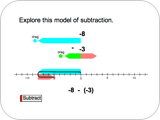Right or Left: Adding and Subtracting Integers

Students add and subtract integers using animations on a number line and understand that subtracting an integer is the same as adding its opposite.

##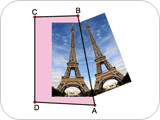Crop and Reflect: Mirror Symmetry

Students create mirror symmetry lines for a variety of pictures, including the Eiffel Tower, a butterfly, letters of the alphabet, and a human face. Optionally, they can import a picture of their own face into Sketchpad and explore its mirror symmetry.

##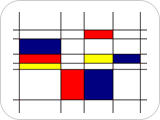Mondrian in Motion: Parallel and Perpendicular Lines

Students construct parallel and perpendicular lines to create virtual artwork in the style of the painter Piet Mondrian. They also explore the properties of two parallelograms, the square and rectangle. As students manipulate their constructions, they change the orientation of the lines and note that parallel or perpendicular lines need not be oriented vertically and horizontally.

##Making a Kaleidoscope: Exploring Rotations

Students create virtual kaleidoscopes by rotating quadrilaterals and then animating them. Students learn that rotated figures keep their size and shape; only their orientation changes. Students make a variety of kaleidoscopes, each with a different number of quadrilaterals and amount of rotation.

##Circles and Squares: Two Unknowns

Students use algebraic thinking as they work with addition statements in which the addends are represented by two symbols. By analyzing the information from two or more statements, students deduce the numerical values of the symbols.